# MCQs on Electromagnetic Fields

##### Page 18 of 20. Go to page 1 2 3 4 5 6 7 8 9 10 11 12 13 14 15 16 17 18 19 20
01․ The energy stored in the magnetic field at a solenoid 30 cm long and 3 cm diameter wound with 1000 turns of wire carrying an current at 10 A, is
0.015 J.
0.15 J.
0.5 J.
1.15 J.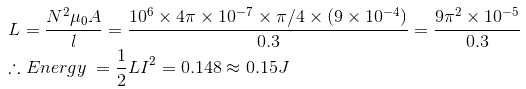02․ A coil of 300 turns is wound on a non-magnetic core having a mean circumference of 300 mm and a cross sectional area of 300 mm2. The current of 3 A will be
37.68 µH.
37.68 mH.
113.04 µH.
113.04 mH.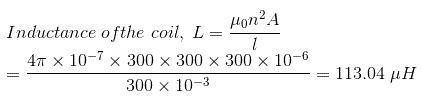03․ A voltage waveform V(t) = 12t2 is applied across a 1H inductor for t = 0, with initial current through it being zero. The current through the inductor for t = 0 is given by
12t.
24t.
12t3.
4t3.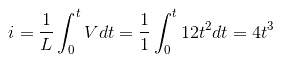04․ Two coupled coils with L1 = L2 = 0.6 H have a coupling coefficient of K = 0.8. The turns ratio N1/N2 is
0.5.
1.
2.
4.

We know the self inductance is given by L = μ0N2A/l; So we see that the self inductance is proportional to the square of the turns in a coil. Hence, L1/L2 = N12/N22; So, N1/N2 = 1.

05․ When a single turn coil rotates in a uniform magnetic field at a uniform speed, the induced emf will be
alternating.
pulsating.
none of these.

The value of induced emf changes per half cycle due to the change in position of the conductor and hence the induced emf is alternating.

06․ The principle of dynamically induced emf is utilized in a
choke.
generator.
transformer.
thermocouple.

In generator emf is induced dynamically as the rate of change of flux is due to the relative motion between magnetic field and conductor.

07․ Two coils are coupled in such a way that the mutual inductance between them is 20 mH. If the inductances of the coils are 40 mH and 50 mH respectively, the coefficient of coupling is
0.447.
0.477.
0.577.
0.01.

The coefficient of coupling is given as K = M/√(L1L2); where M is the mutual inductance, L1 and L2 are the self inductances respectively So,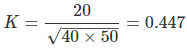08․ The two windings of a transformer have an inductor of 2 H each. If mutual inductor between them is also 2 H, then
turns ratio of the transformer is also two.
transformer is an ideal transformer.
it is a perfect transformer.
none of these.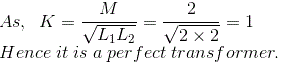09․ What is the magnetomotive force in a 75-turn coil of wire when there are 4 A of current through it?
18.75.
150.
300.
1200.

MMF = N × I; MMF = 4 × 75 = 300.

10․ Magnetostriction is a phenomenon of
generation of electricity in ferromagnetic materials.
change in physical dimensions of ferromagnetic materials during magnetism.
change in permeability of ferromagnetic materials during magnetism.
generation of magnetism in electrical conductors.

Magnetostriction is a property of ferromagnetic materials that causes them to change their shape or dimensions during the process of magnetization. The variation of materials' magnetization due to the applied magnetic field changes the magnetostrictive strain until reaching its saturation value. The effect was first identified by James Joule. This effect causes energy loss due to frictional heating in susceptible ferromagnetic cores. The effect is also responsible for the low-pitched humming sound that can be heard coming from transformers, where oscillating AC currents produce a changing magnetic field.

<<<1617181920>>>